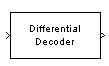# Differential Decoder

Decode binary signal using differential coding

## Library

Source Coding

•## Description

The Differential Decoder block decodes the binary input signal. The output is the logical difference between the consecutive input element within a channel. More specifically, the block's input and output are related by

m(i0) = d(i0) XOR Initial condition parameter value

m(ik) = d(ik) XOR d(ik-1)

where

• d is the differentially encoded input.

• m is the output message.

• ik is the kth element.

• XOR is the logical exclusive-or operator.

This block accepts a scalar, column vector, or matrix input signal and treats columns as channels.

## Parameters

Initial conditions

The logical exclusive-or of this value with the initial input value forms the initial output value.

## Supported Data Type

PortSupported Data Types
In
• double

• single

• boolean

• integer

• fixed-point

Out
• double

• single

• boolean

• integer

• fixed-point

## References

 Couch, Leon W., II, Digital and Analog Communication Systems, Sixth edition, Upper Saddle River, N. J., Prentice Hall, 2001.

## Pair Block

Differential Encoder

## Version History

Introduced before R2006a